Shared cart
Track an order
Reward not redeemed
You dont have enough points to redeem this reward.
Favorite list
Alternative Items

## Shop all Union & Scale (858 products)

Filter Your Categories 858 items found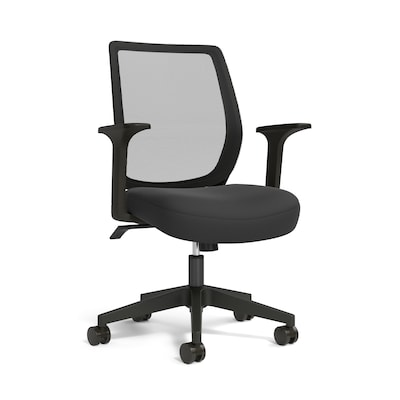\$179.99 Save 44%
\$99.99
Per each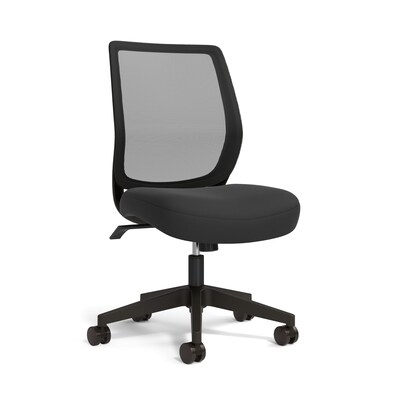\$149.99
Per each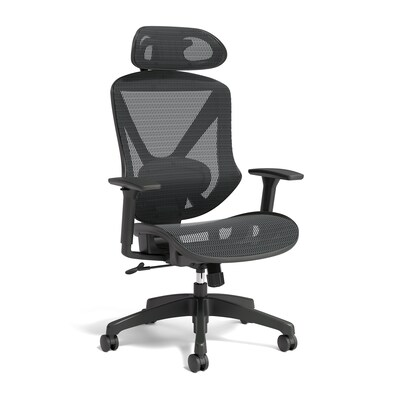\$299.99 Save 33%
\$199.99
Per each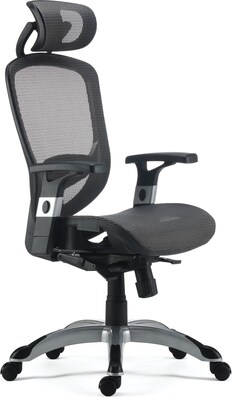\$309.99 Save 35%
\$199.99
Per each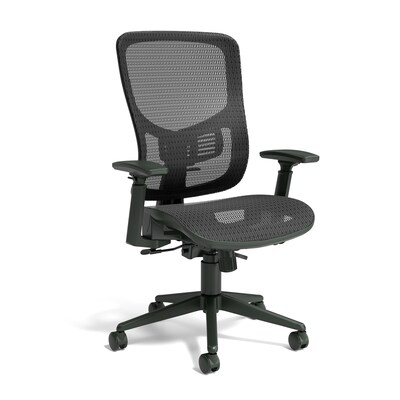\$259.99 Save 23%
\$199.99
Per each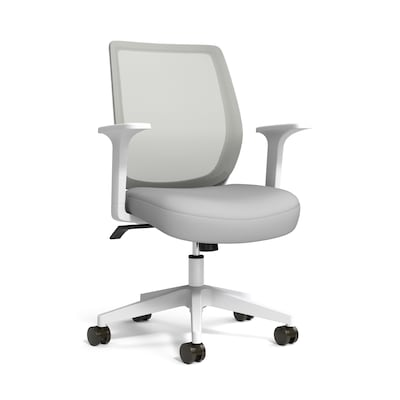\$179.99 Save 38%
\$109.99
Per each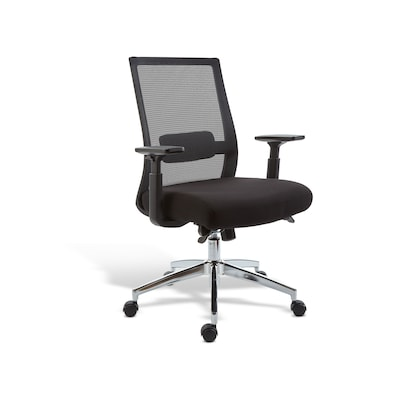\$249.99
Per each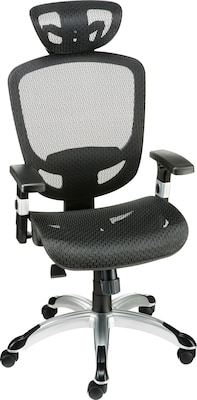\$309.99 Save 29%
\$219.99
Per each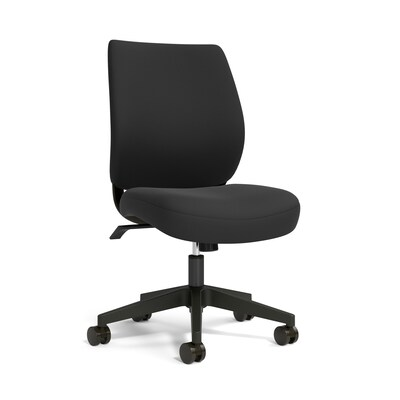\$149.99 Save 26%
\$109.99
Per each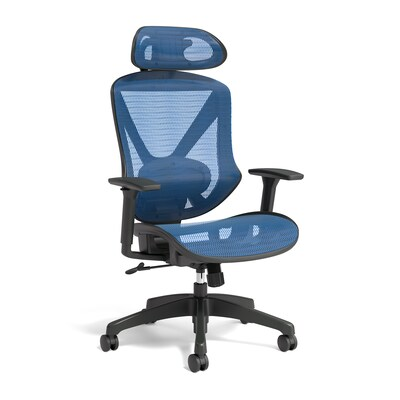\$299.99 Save 36%
\$189.99
Per each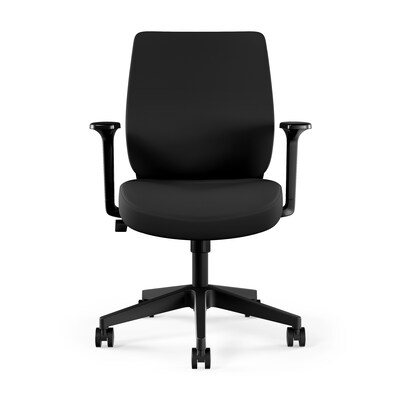\$159.99
Per each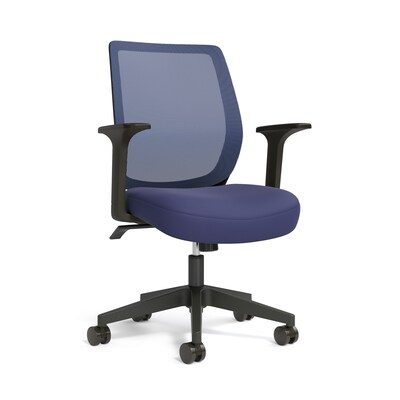\$179.99
Per each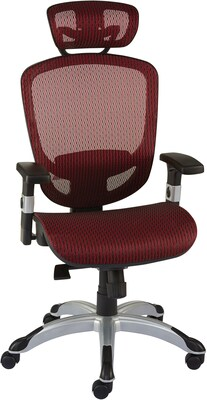\$309.99 Save 29%
\$219.99
Per each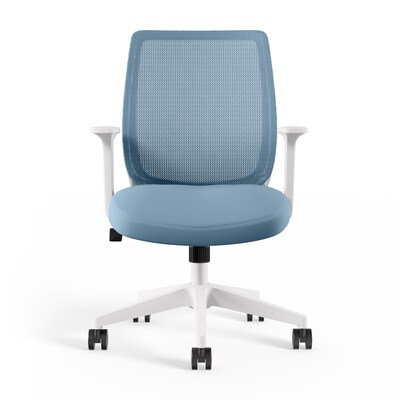\$179.99 Save 38%
\$109.99
Per each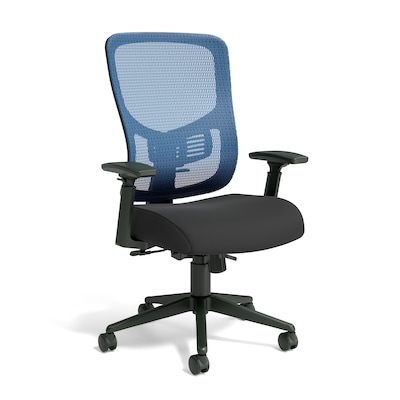\$219.99 Save 22%
\$169.99
Per each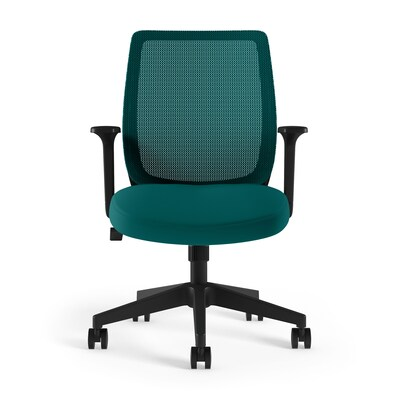\$179.99
Per each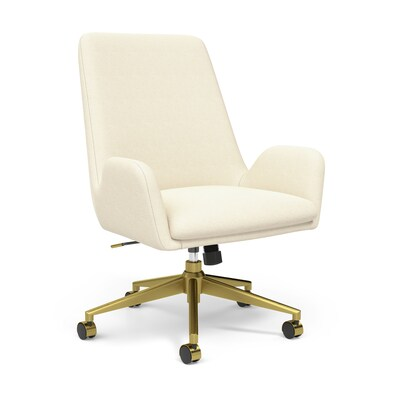\$239.99 Save 25%
\$179.99
Per each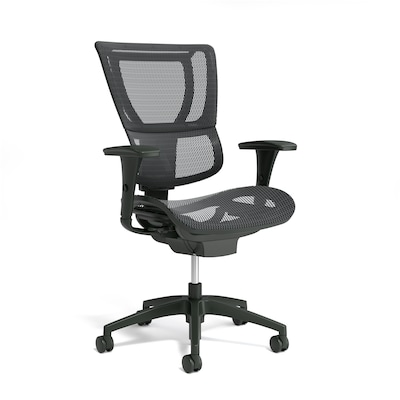\$559.99
Per each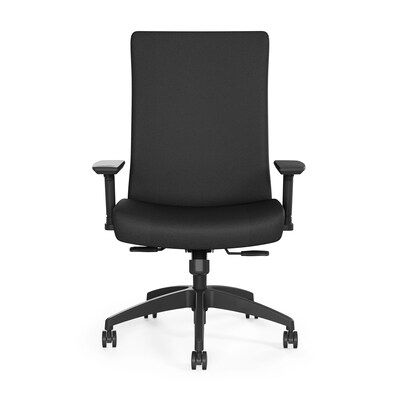\$319.99
Per each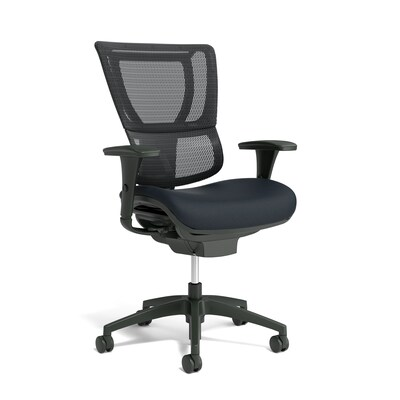\$559.99
Per each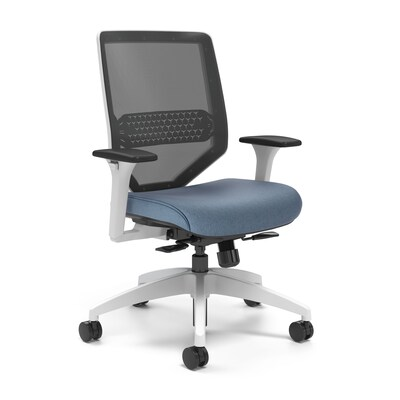\$499.99
Per each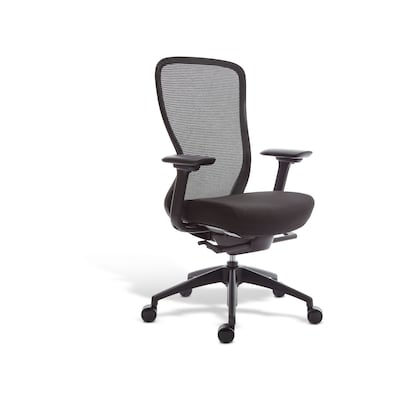\$399.99
Per each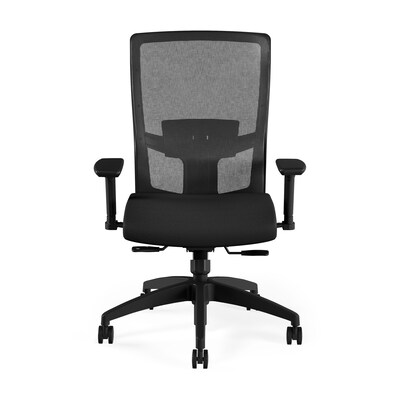\$429.99
Per each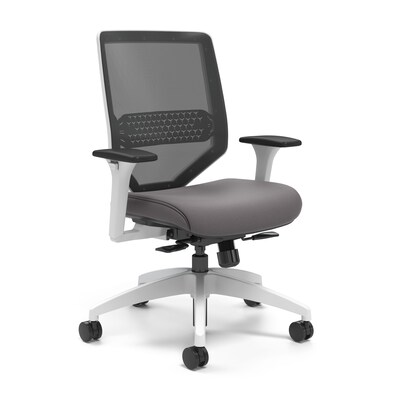\$499.99
Per each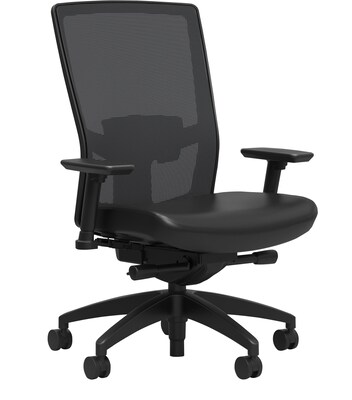\$369.99
Per each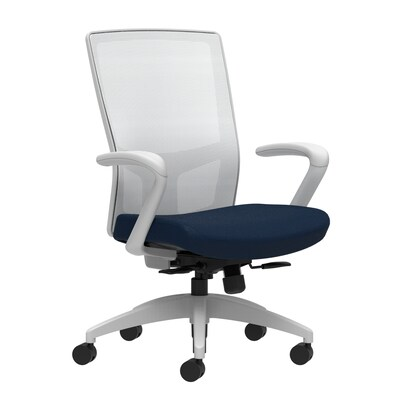\$489.99
Per each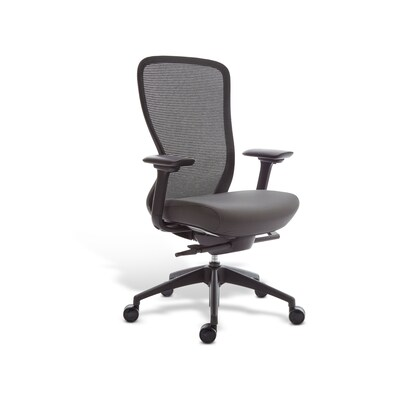\$379.99
Per each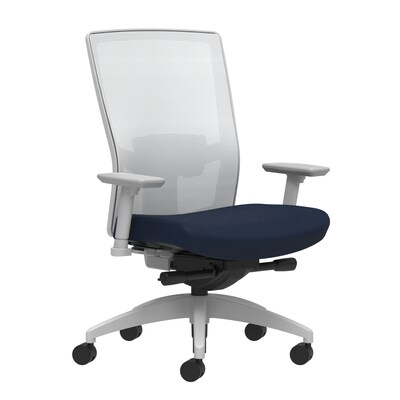\$569.99
Per each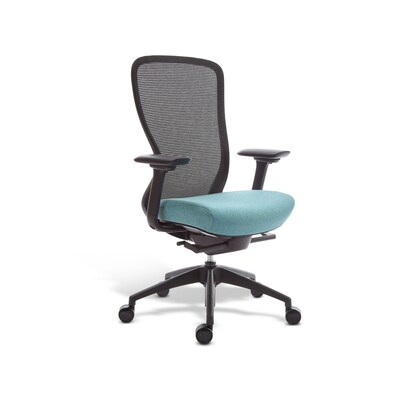\$389.99
Per each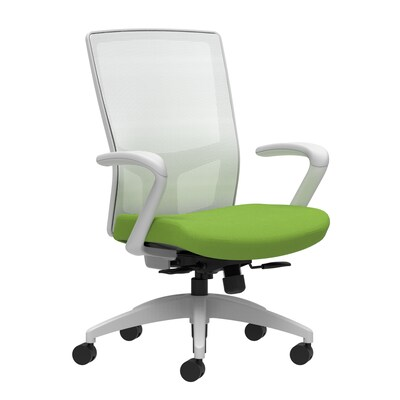\$459.99
Per each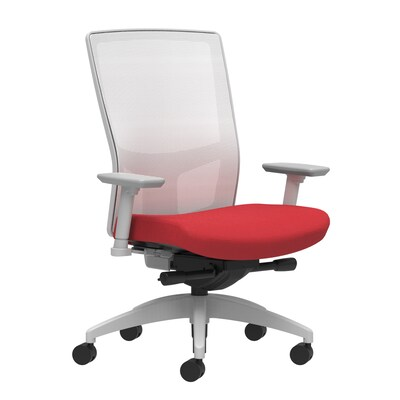\$499.99
Per each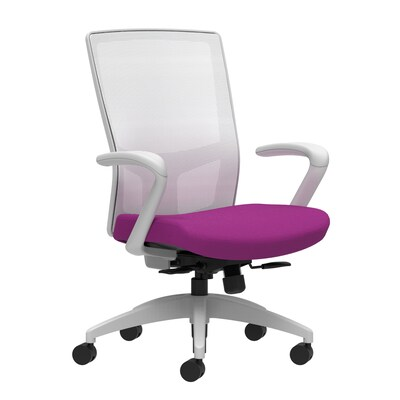\$459.99
Per each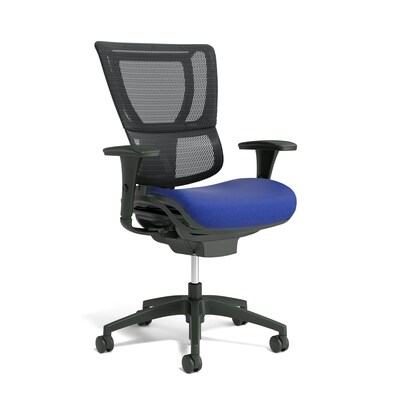\$559.99
Per each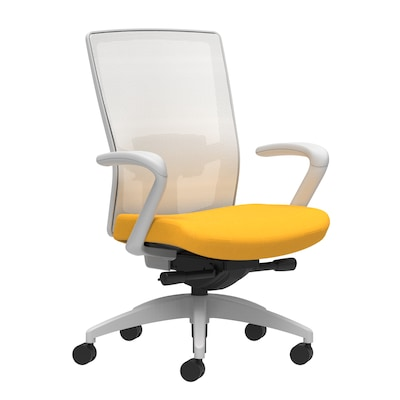\$549.99
Per each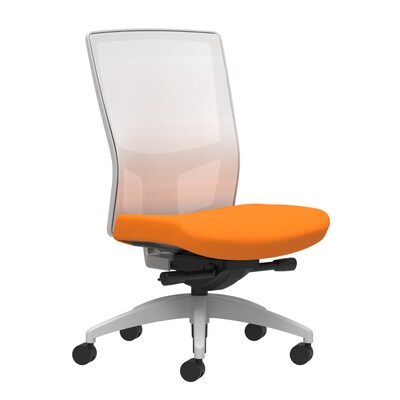\$504.99
Per each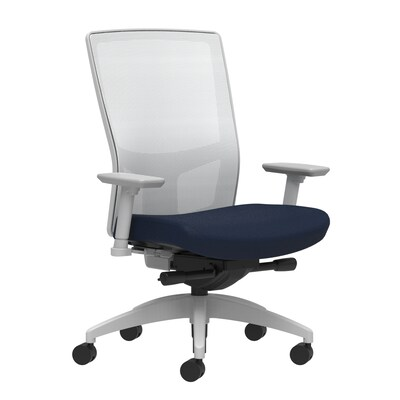\$499.99
Per each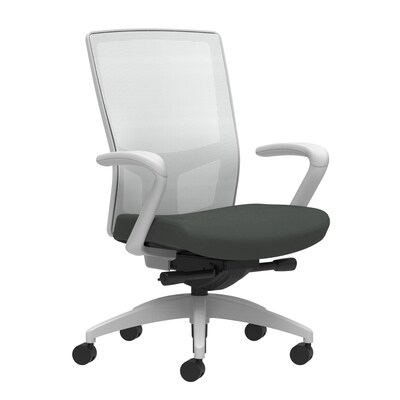\$534.99
Per each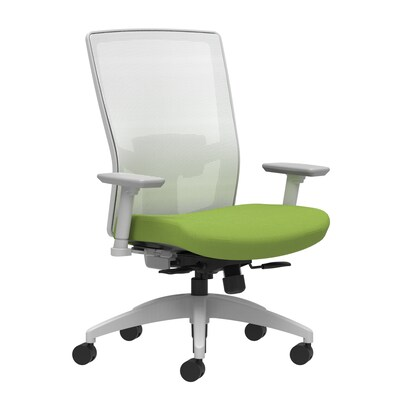\$544.99
Per each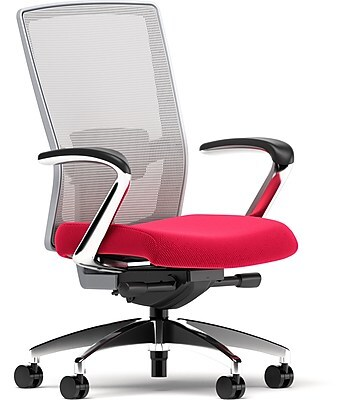\$619.99
Per each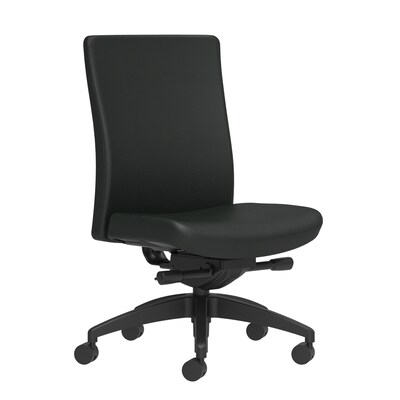\$469.99
Per each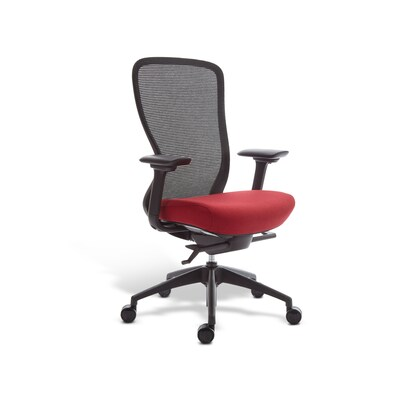\$404.99
Per each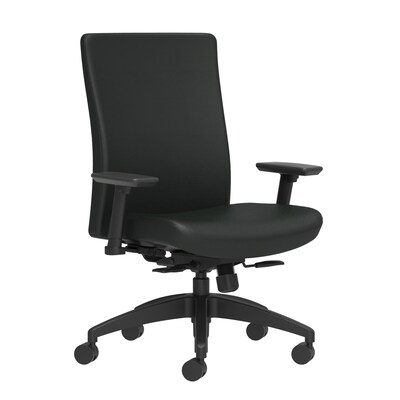\$509.99
Per each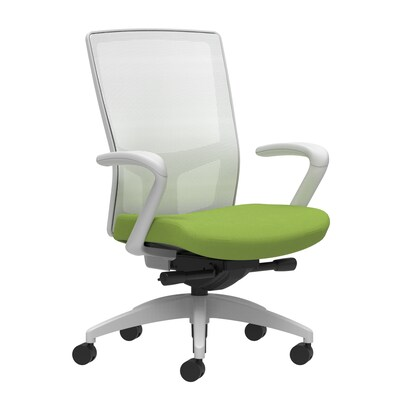\$534.99
Per each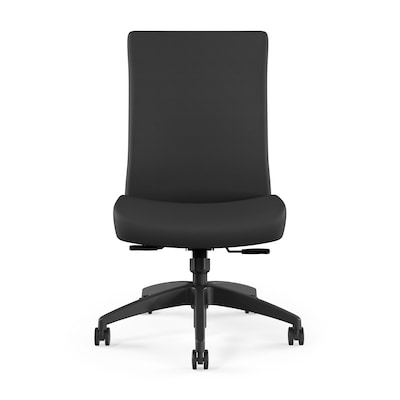\$429.99
Per each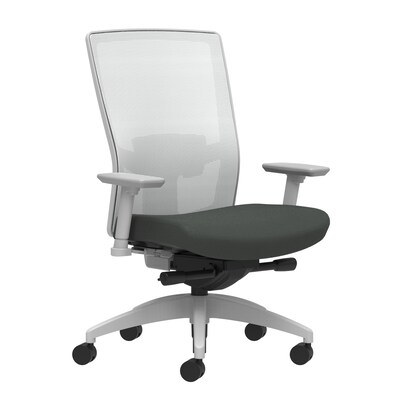\$569.99
Per each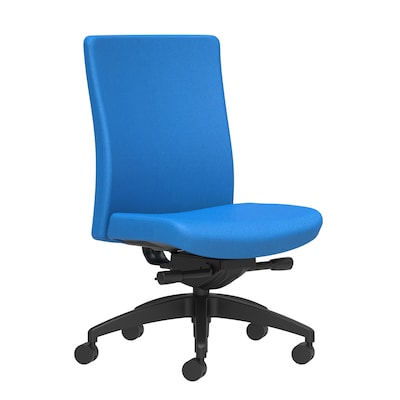\$489.99
Per each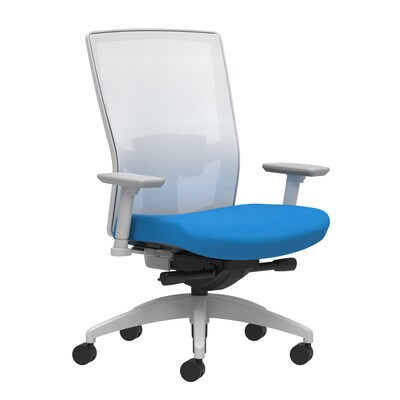\$569.99
Per each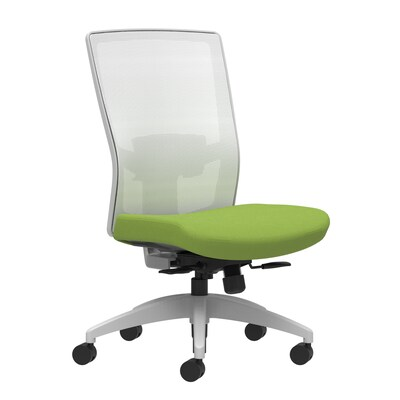\$469.99
Per each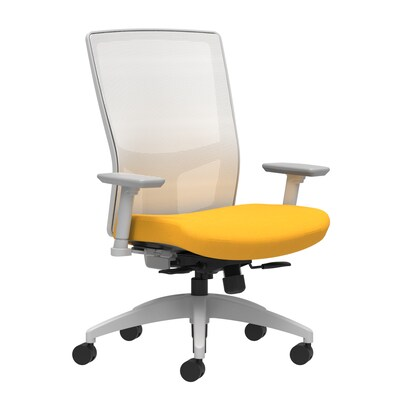\$474.99
Per each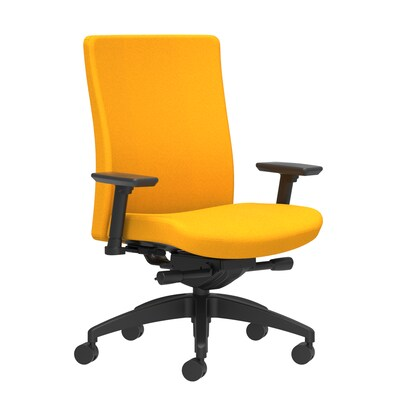\$539.99
Per each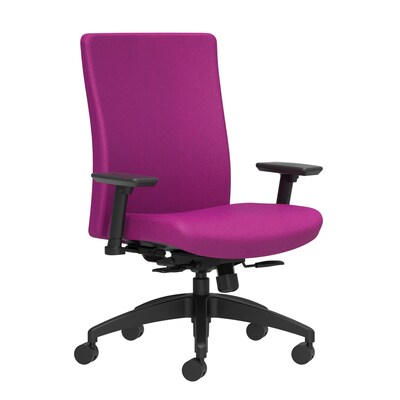\$494.99
Per each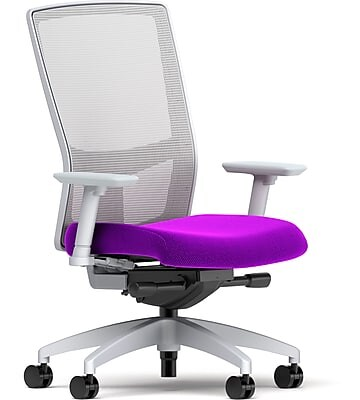\$499.99
Per each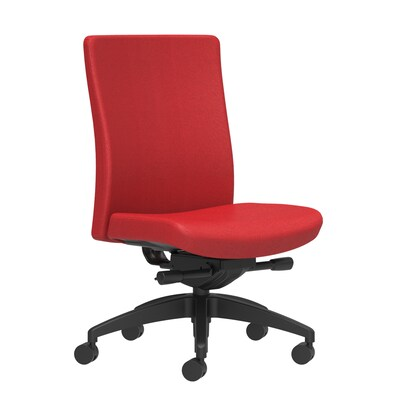\$489.99
Per each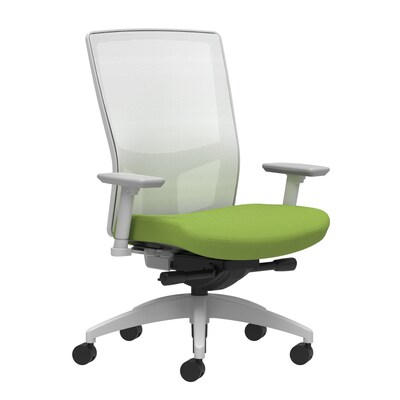\$499.99
Per each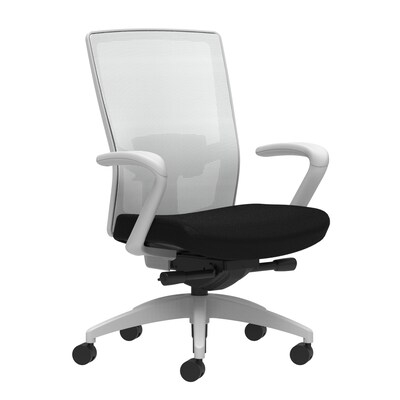\$549.99
Per each